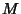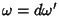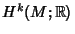## de Rham Cohomology

de Rham cohomology is a formal set-up for the analytic problem: If you have a Differential k-Formon a Manifold, is it the Exterior Derivative of another Differential k-Form? Formally, ifthen. This is more commonly stated as, meaning that ifis to be the Exterior Derivative of a Differential k-Form, a Necessary condition thatmust satisfy is that its Exterior Derivative is zero.

de Rham cohomology gives a formalism that aims to answer the question, Are all differential-forms on a Manifold with zero Exterior Derivative the Exterior Derivatives of-forms?'' In particular, theth de Rham cohomology vector space is defined to be the space of all-forms with Exterior Derivative 0, modulo the space of all boundaries of-forms. This is the trivial Vector Space Iff the answer to our question is yes.

The fundamental result about de Rham cohomology is that it is a topological invariant of the Manifold, namely: theth de Rham cohomology Vector Space of a Manifoldis canonically isomorphic to the Alexander-Spanier Cohomology Vector Space(also called cohomology with compact support). In the case thatis compact, Alexander-Spanier Cohomology is exactly singular cohomology.

See also Alexander-Spanier Cohomology, Change of Variables Theorem, Differential k-Form, Exterior Derivative, Vector Space